Produces a density estimate for each data point using a Gaussian finite mixture model from Mclust.

densityMclust(data, ...)

## Arguments

data A numeric vector, matrix, or data frame of observations. Categorical variables are not allowed. If a matrix or data frame, rows correspond to observations and columns correspond to variables. Additional arguments for the Mclust function. In particular, setting the arguments G and modelNames allow to specify the number of mixture components and the type of model to be fitted. By default an "optimal" model is selected based on the BIC criterion.

## Value

An object of class densityMclust, which inherits from Mclust, is returned with the following slot added:

density

The density evaluated at the input data computed from the estimated model.

Scrucca L., Fop M., Murphy T. B. and Raftery A. E. (2016) mclust 5: clustering, classification and density estimation using Gaussian finite mixture models, The R Journal, 8/1, pp. 289-317.

Fraley C. and Raftery A. E. (2002) Model-based clustering, discriminant analysis and density estimation, Journal of the American Statistical Association, 97/458, pp. 611-631.

Fraley C., Raftery A. E., Murphy T. B. and Scrucca L. (2012) mclust Version 4 for R: Normal Mixture Modeling for Model-Based Clustering, Classification, and Density Estimation. Technical Report No. 597, Department of Statistics, University of Washington.

## Author

Revised version by Luca Scrucca based on the original code by C. Fraley and A.E. Raftery.

plot.densityMclust, Mclust, summary.Mclust, predict.densityMclust.

## Examples

dens <- densityMclust(faithful$waiting) summary(dens) #> ------------------------------------------------------- #> Density estimation via Gaussian finite mixture modeling #> ------------------------------------------------------- #> #> Mclust E (univariate, equal variance) model with 2 components: #> #> log-likelihood n df BIC ICL #> -1034.002 272 4 -2090.427 -2099.576summary(dens, parameters = TRUE) #> ------------------------------------------------------- #> Density estimation via Gaussian finite mixture modeling #> ------------------------------------------------------- #> #> Mclust E (univariate, equal variance) model with 2 components: #> #> log-likelihood n df BIC ICL #> -1034.002 272 4 -2090.427 -2099.576 #> #> Mixing probabilities: #> 1 2 #> 0.3609461 0.6390539 #> #> Means: #> 1 2 #> 54.61675 80.09239 #> #> Variances: #> 1 2 #> 34.44093 34.44093 plot(dens, what = "BIC", legendArgs = list(x = "topright"))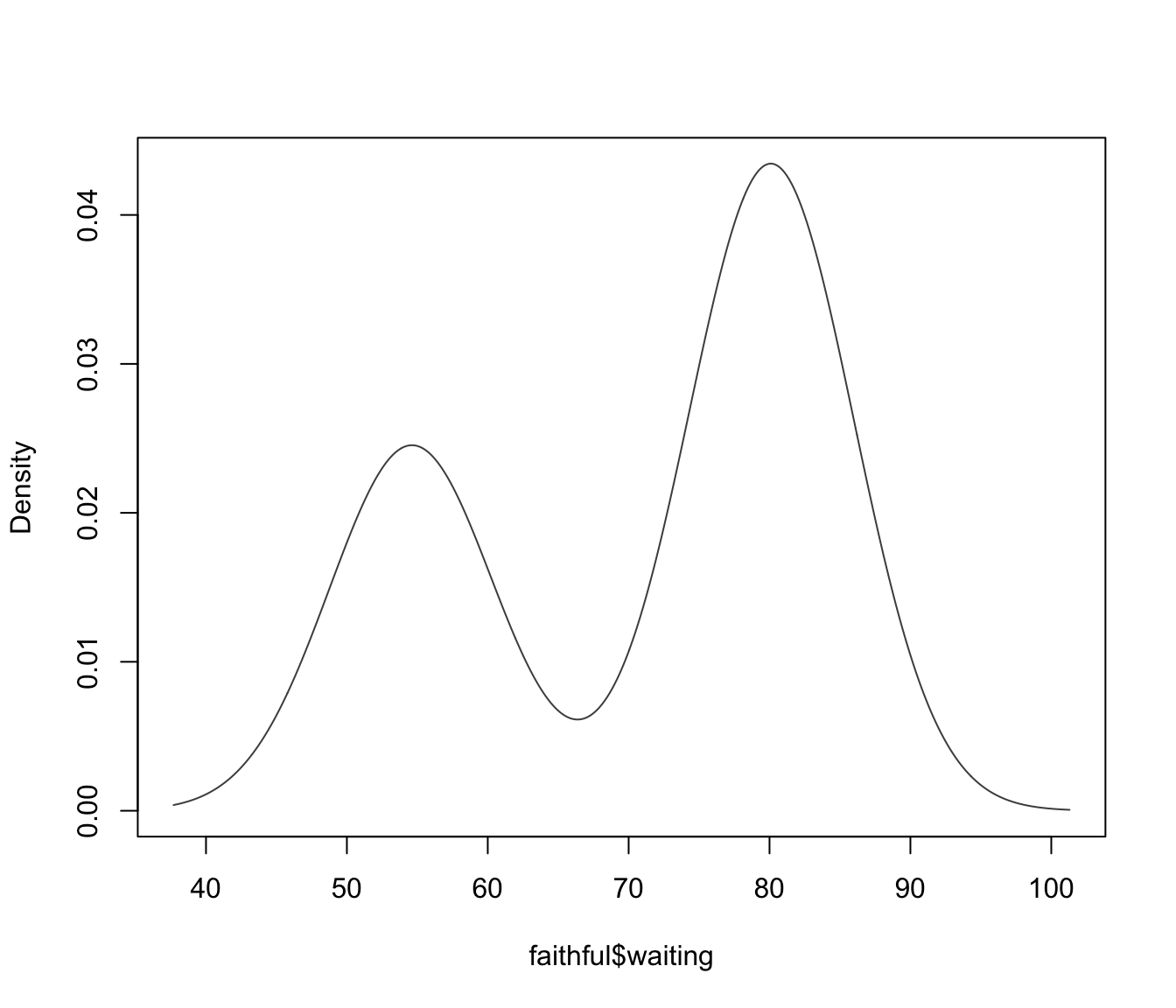plot(dens, what = "density", data = faithful$waiting)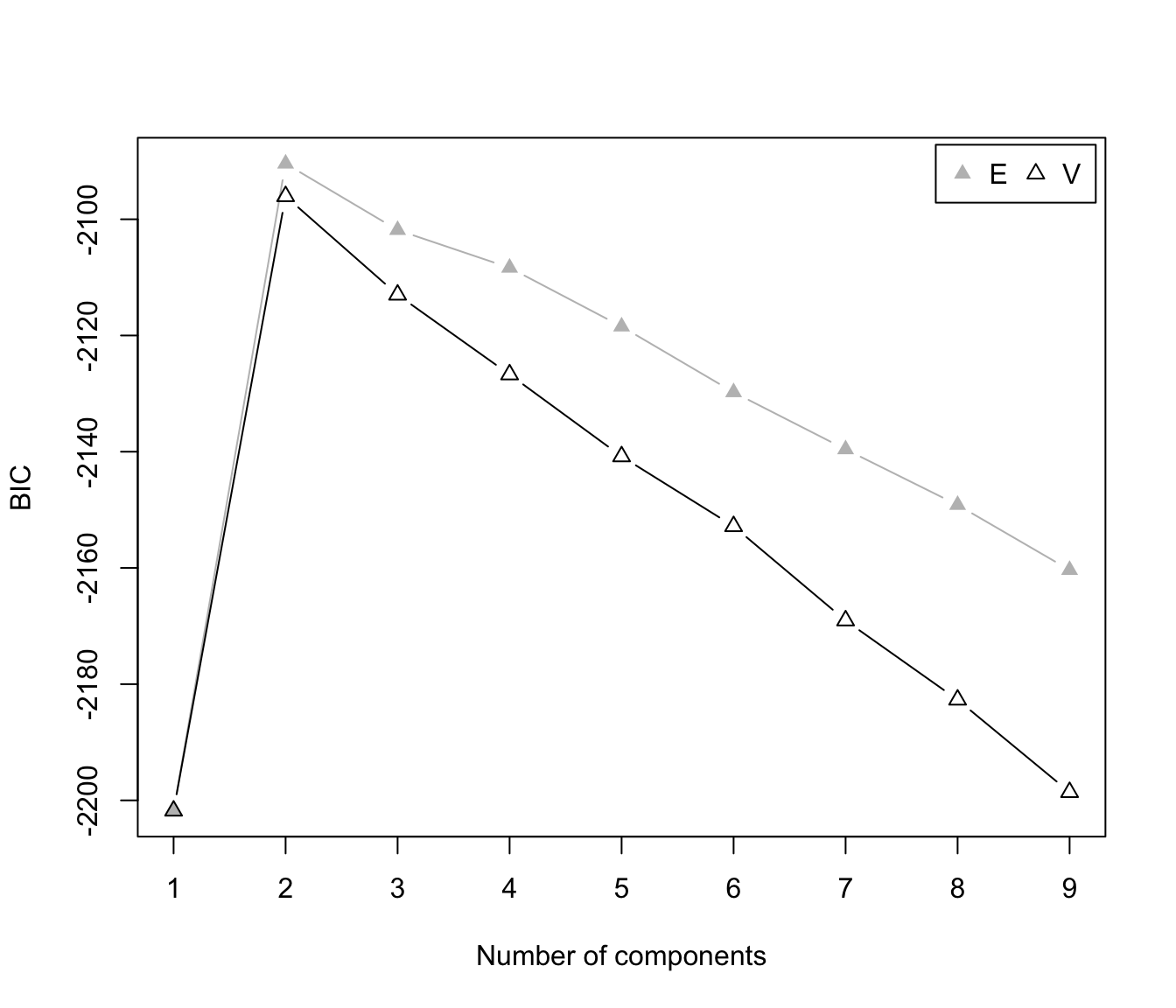dens <- densityMclust(faithful, modelNames = "EEE", G = 3)
summary(dens)
#> -------------------------------------------------------
#> Density estimation via Gaussian finite mixture modeling
#> -------------------------------------------------------
#>
#> Mclust EEE (ellipsoidal, equal volume, shape and orientation) model with 3
#> components:
#>
#>  log-likelihood   n df       BIC       ICL
#>       -1126.326 272 11 -2314.316 -2357.824summary(dens, parameters = TRUE)
#> -------------------------------------------------------
#> Density estimation via Gaussian finite mixture modeling
#> -------------------------------------------------------
#>
#> Mclust EEE (ellipsoidal, equal volume, shape and orientation) model with 3
#> components:
#>
#>  log-likelihood   n df       BIC       ICL
#>       -1126.326 272 11 -2314.316 -2357.824
#>
#> Mixing probabilities:
#>         1         2         3
#> 0.1656784 0.3563696 0.4779520
#>
#> Means:
#>                [,1]      [,2]      [,3]
#> eruptions  3.793066  2.037596  4.463245
#> waiting   77.521051 54.491158 80.833439
#>
#> Variances:
#> [,,1]
#>            eruptions    waiting
#> eruptions 0.07825448  0.4801979
#> waiting   0.48019785 33.7671464
#> [,,2]
#>            eruptions    waiting
#> eruptions 0.07825448  0.4801979
#> waiting   0.48019785 33.7671464
#> [,,3]
#>            eruptions    waiting
#> eruptions 0.07825448  0.4801979
#> waiting   0.48019785 33.7671464plot(dens, what = "density", data = faithful,
drawlabels = FALSE, points.pch = 20)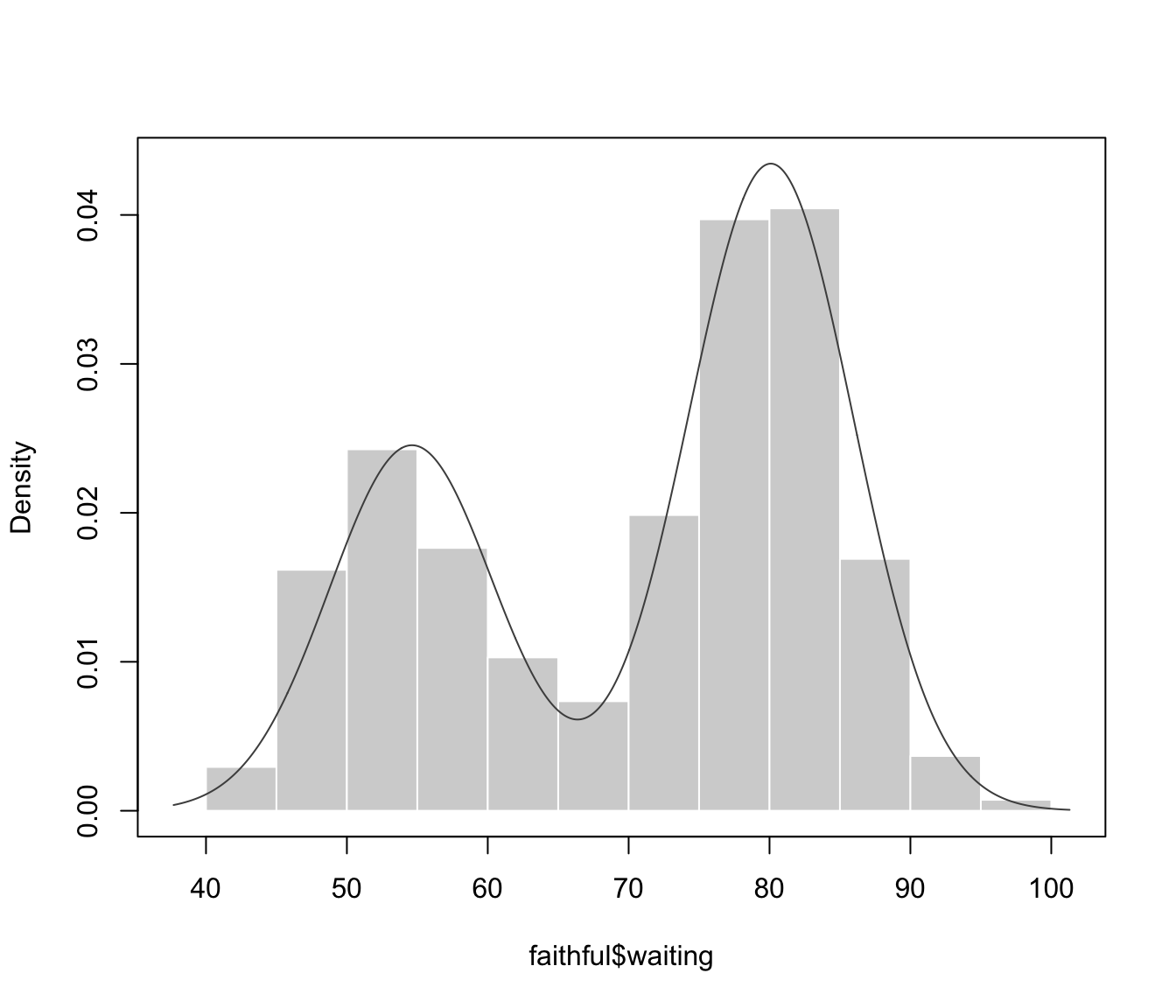plot(dens, what = "density", type = "hdr")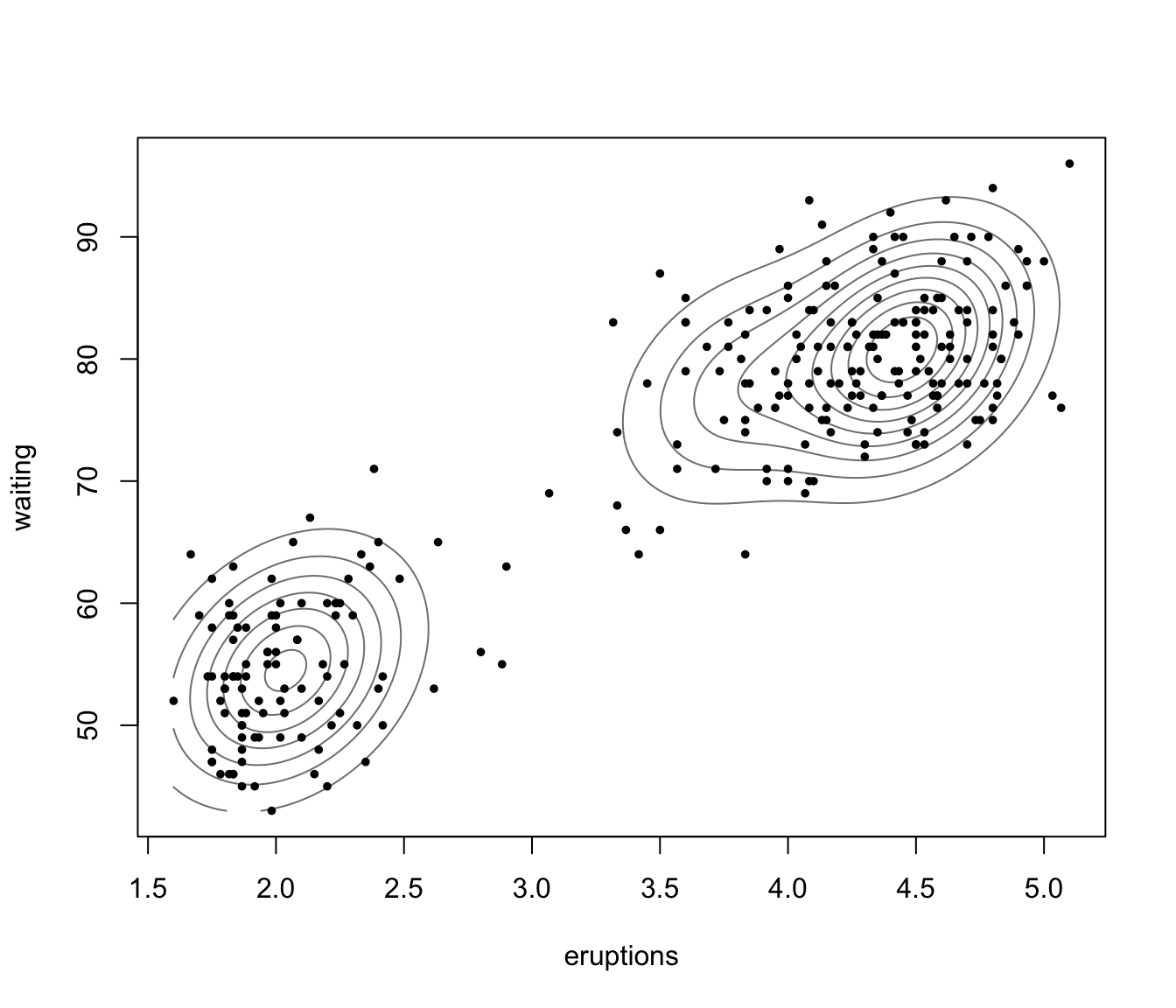plot(dens, what = "density", type = "hdr", prob = c(0.1, 0.9))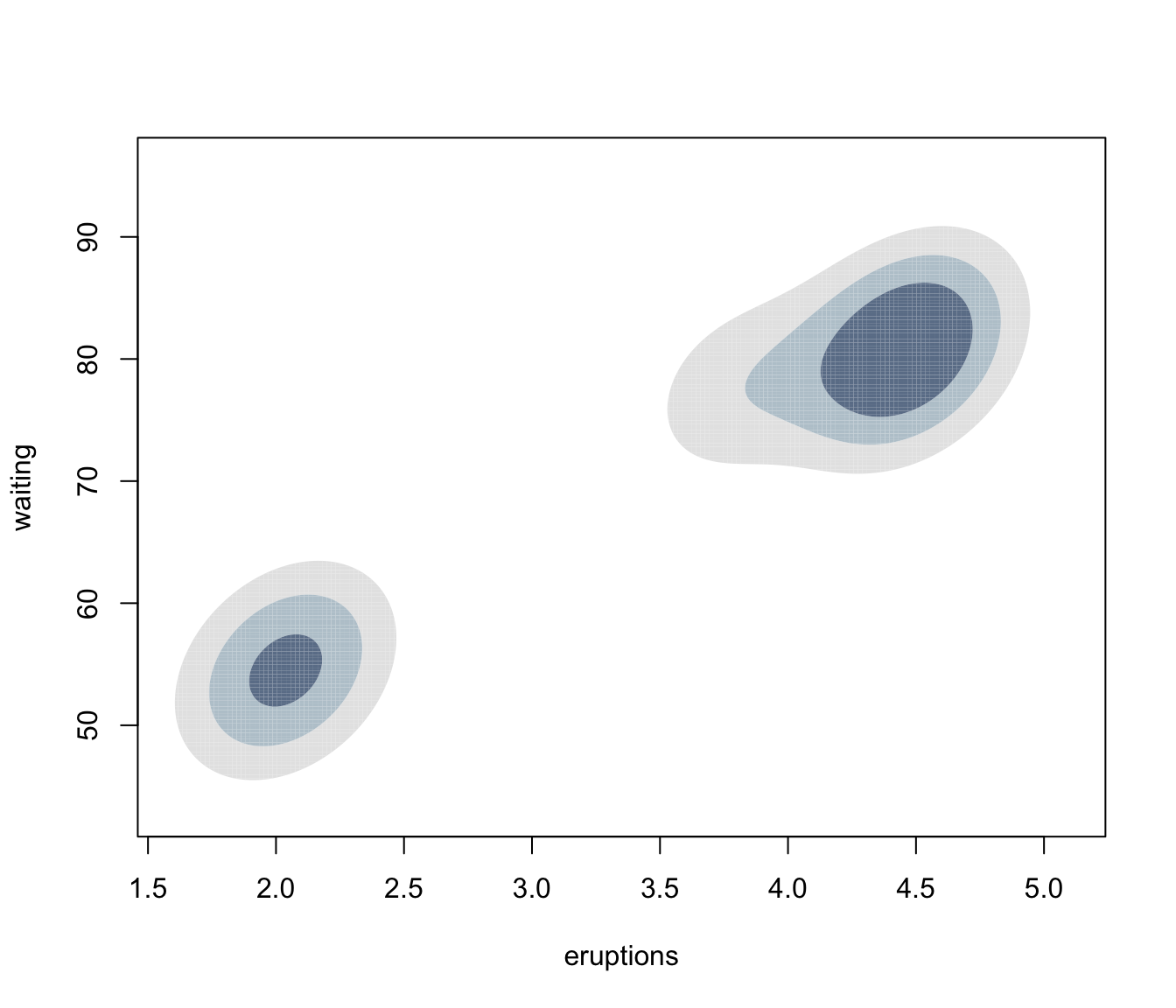plot(dens, what = "density", type = "hdr", data = faithful)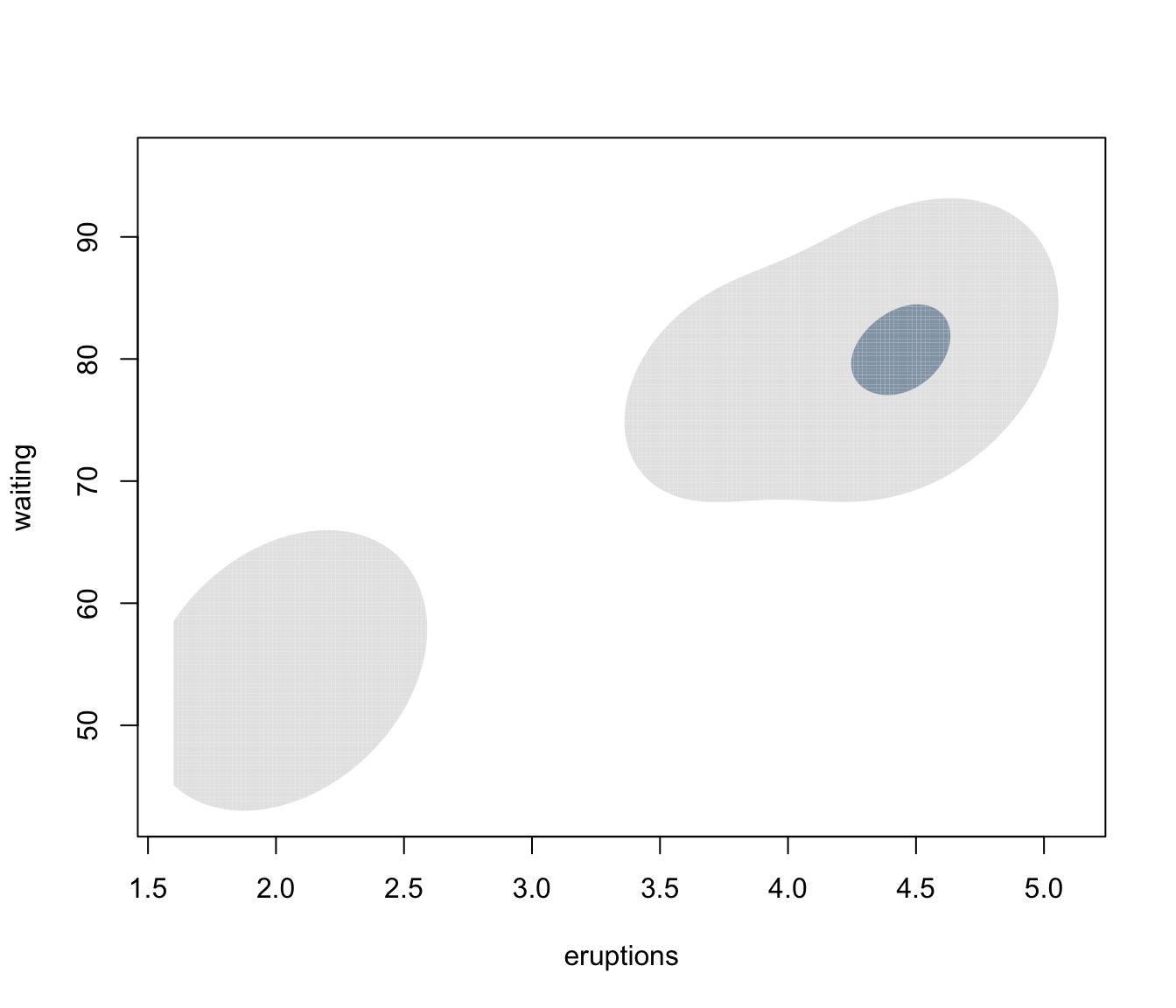plot(dens, what = "density", type = "persp")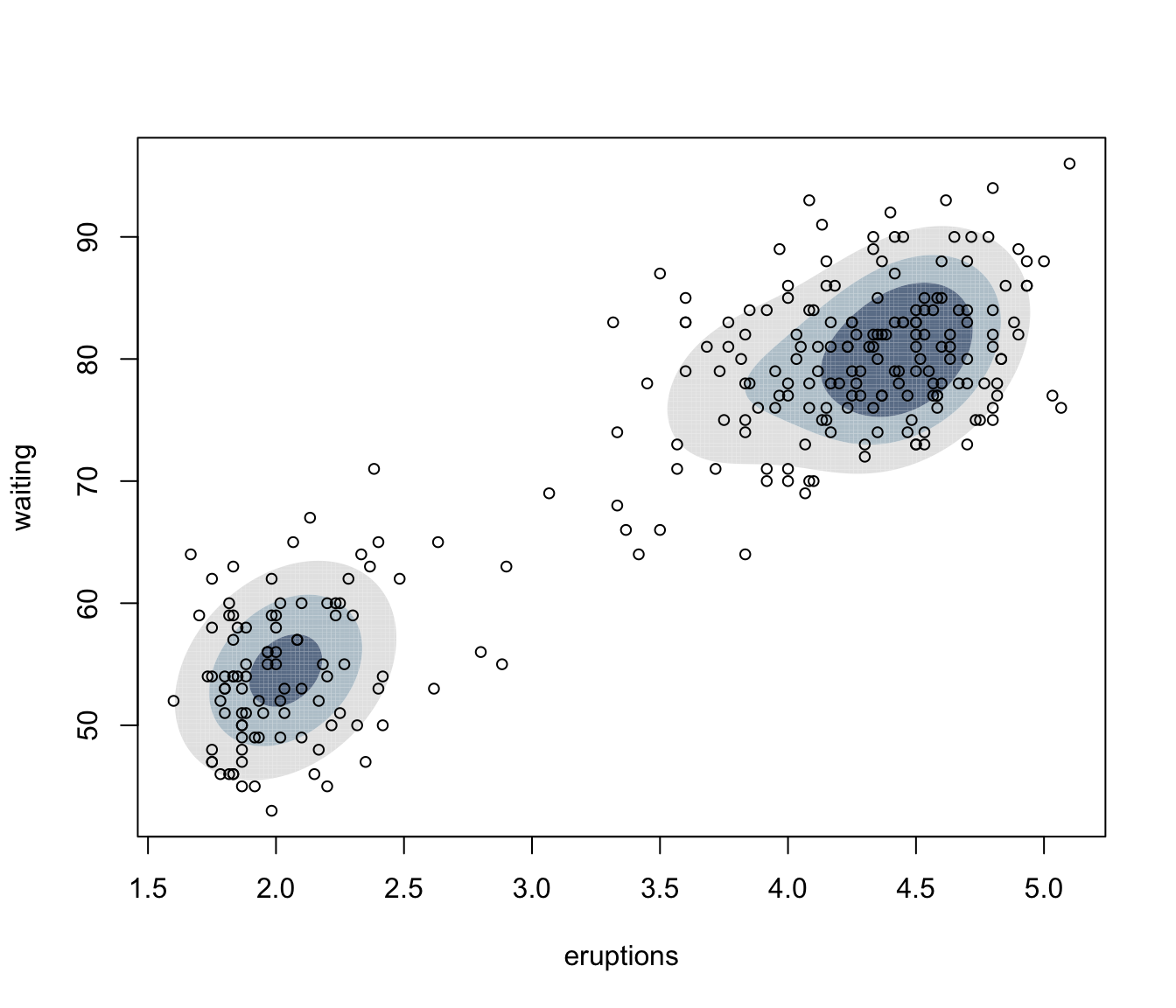if (FALSE) {
dens <- densityMclust(iris[,1:4], G = 2)
summary(dens, parameters = TRUE)
plot(dens, what = "density", data = iris[,1:4],
col = "slategrey", drawlabels = FALSE, nlevels = 7)
plot(dens, what = "density", type = "hdr", data = iris[,1:4])
plot(dens, what = "density", type = "persp", col = grey(0.9))
}